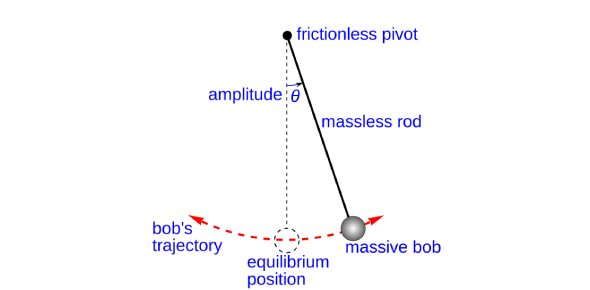# Physics: Trivia Questions On Pendulum! Quiz

7 Questions | Total Attempts: 205Settings.

• 1.
Pendulums have bobs and rods and pivrets
• A.

True

• B.

False

• 2.
The mass at the bottom of the pendulum is.......
• A.

The rod

• B.

The pivot

• C.

The bob

• D.

The inertia

• 3.
A period is half a pendulum beat
• A.

True

• B.

False

• 4.
The bob is a timer that counts the number of beats or periods for you
• A.

True

• B.

False

• 5.
Inertia is the law that___________________________
• 6.
Pendulums are easy to make if you have the right stuff.
• A.

True

• B.

False

Related Topics